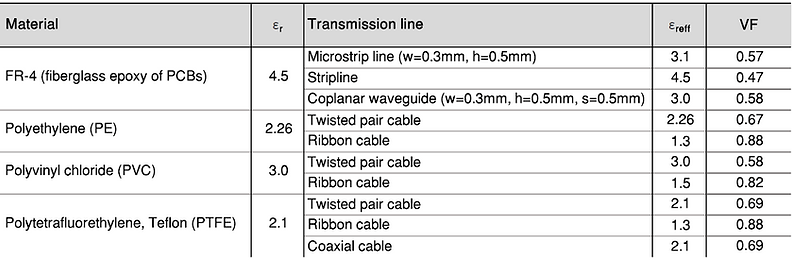top of page

## 2  Frequency & Wavelength

It is fundamental that professional engineers in the field of EMC understand the basics of signal frequency f [Hz] and wavelength λ [m]:

EMC and Frequency.

In general, EMC issues occur with signals of frequency > 9kHz. This is the reason why most EMC Standards do not consider signals with < 9kHz.

• Conducted Emission. Conducted emissions tend to occur at f < 30MHz.

• Radiated Emission. Radiated emissions tend to occur at f > 30MHz.

Wavelength: Calculation.

The frequency f of a sinusoidal signal and its wavelength λ [m] have the following relationship [2.1]:where v [m/sec] is the propagation velocity of the signal and f [Hz] is the frequency of the signal.Wavelength in any media.

Transverse electromagnetic (TEM) is a mode of propagation where the electric and magnetic fields are all restricted to directions normal (transverse) to the direction of propagation (neither electric nor magnetic field in the direction of propagation). Plane waves are TEM waves.

The frequency f [Hz] of a sinusoidal electromagnetic TEM wave and its wavelength λ [m] have the following relationship [2.5]:where v [m/sec] is the propagation velocity of the signal, f [Hz] is the frequency of the signal, β [1/m] is the propagation constant of the media, ε' [F/m] is the real part of the complex permittivity ε'+jε'', ε'' [F/m] is the imaginary part of the complex permittivity ε=ε'+jε'', µ' [H/m] is the real part of the complex permeability µ'+jµ''µ'' [H/m] is the imaginary part of the complex permeability µ'+jµ''.

Wavelength in insulating media.

In the case that an electromagnetic wave travels through an insulator (μr'= 1) with negligible dielectric and magnetic losses (ε''=0, μ''=0), the wavelength λ [m] of such a sinusoidal electromagnetic wave with frequency f [Hz] can be written as [2.5]:where v [m/sec] is the propagation velocity of the signal, f [Hz] is the frequency of the signal, c [m/sec] is the speed of light (2.998·10^8m/sec), ε0 [F/m] is the permittivity of vacuum (8.854·10^−12 F/m), µ0 [H/m] is the permeability of vacuum (12.57·10^−7 H/m) and εr' is the relative permittivity (dielectric constant of the insulator).

Wavelength in vacuum.

In the case that an electromagnetic wave travels through vacuum (and approximately air), the wavelength λ [m] of such a sinusoidal electromagnetic wave with frequency f [Hz] can be written as [2.5]:where c [m/sec] is the speed of light (2.998·10^8m/sec), ε0 [F/m] is the permittivity of vacuum (8.854·10^−12 F/m), µ0 [H/m] is the permeability of vacuum (12.57·10^−7 H/m).

Wavelength in good conducting media (e.g. shield).

In the case that an electromagnetic sinusoidal wave travels through a good conductor
(through and not along(!), e.g. through a shield) with negligible magnetic losses
(µ'' = 0), the wavelength can be calculated as [2.5]:where f [Hz] is the frequency of the sinusoidal signal, µ'=µr0 [H/m] is the real part of the complex permeability (µ=µ'-jµ'') and σ [S/m] is the specific conductance of the medium where the wave is propagating through.

Wavelength of signals traveling long blank wires vs. cables & PCB traces.

It is important to understand that the signal propagation velocity v [m/sec] depends on the transport medium through which the electromagnetic field is traveling. Therefore, the same signal with the same frequency f [Hz] has a different wavelength λ [m] in a blank wire (surrounded by air) than in a cable or PCB trace (surrounded by insulation material).​ The wavelength λ [m] is calculated the following way [2.1]:where v is the signal propagation velocity in [m/sec], c is the speed of light (3E8 [m/sec]), f is the frequency of the sinusoidal signal in [Hz], εr is the relative permittivity and μr is the relative permeability of the media through which the electromagnetic field is propagating. VF is called the velocity factor.

• Wavelength in a blank wire. The wavelength λ of a signal with frequency f along a blank wire (or antenna surrounded by air) depends only on the speed of light c and the signal frequency f (v=c, because εr = 1 and μr =1 and therefore VF=1) [2.1]:• Wavelength in cables and PCB traces. The wavelength λ of a signal with frequency f along an insulated copper wire or a cable or a Printed Circuit Board (PCB) trace is [2.1]:Where c is the speed of light (3E8 [m/sec]), f is the signal frequency [Hz], εreff the effective dielectric constant (relative permittivity) through which the electromagnetic wave is propagating. The effective dielectric constant εreff is defined as the uniform equivalent dielectric constant for a transmission line, even in presence of different dielectrics (e.g. FR-4 and air for a microstrip line, see picture below).
The relative permeability μr is assumed to be equal to 1.0 for cables and PCBs because the insulation materials are non-magnetic. Thus, the velocity factor VF depends primarily on the effective relative permittivity (also called effective dielectric constant) εreff of the insulation or PCB material.

The calculation of the effective dielectric constant εreff  depends on the insulation material and the geometry of the transmission line (e.g. ribbon cable, microstrip, coplanar waveguide, etc.), because the amount of the electric field lines in the different media depending on the geometry of the transmission line (e.g. see the microstrip line below).The Excel sheet below contains a calculator for calculating the effective dielectric constant εreff  (effective permittivity) for some of the most common transmission lines:

• PCB-traces. Microstrip, stripline, coplanar waveguide with a reference plane.

• Cables. Ribbon cable, twisted pair.

The velocity factor VF  of a transmission medium is the ratio of the velocity v [m/sec] at which a wavefront of an electromagnetic signal passes through the medium, compared to the speed of light in vacuum c [3E8m/sec]: VF=v/c. Thus, the smaller the velocity factor VF , the smaller the wavelength λ [m]. The table below shows the approximate velocity factors for different insulation and PCB materials and different transmission line types [2.2, 2.3, 2.4].The table below shows some rough approximations of wavelengths in different conductors (cables, PCBs) compared to free-space (air). Possible assumptions for wavelength λ in PCBs (FR-4) and cables are:

• PCB. λPCB≈0.5*λair (assumption εreff ≈3.0...4.5 → VF≈0.5).

• Cables. λCable≈0.7*λair (assumption εreff ≈1.5...3.0 → VF≈0.7).EMC and Frequency
Wavelengh Calculation
Wavelength Blank Wire vs. Cable
bottom of page